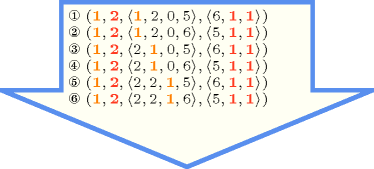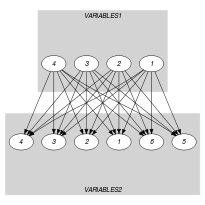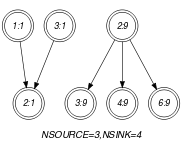## 5.75. common

Origin

N. Beldiceanu

Constraint

$\mathrm{𝚌𝚘𝚖𝚖𝚘𝚗}\left(\mathrm{𝙽𝙲𝙾𝙼𝙼𝙾𝙽}\mathtt{1},\mathrm{𝙽𝙲𝙾𝙼𝙼𝙾𝙽}\mathtt{2},\mathrm{𝚅𝙰𝚁𝙸𝙰𝙱𝙻𝙴𝚂}\mathtt{1},\mathrm{𝚅𝙰𝚁𝙸𝙰𝙱𝙻𝙴𝚂}\mathtt{2}\right)$

Arguments
 $\mathrm{𝙽𝙲𝙾𝙼𝙼𝙾𝙽}\mathtt{1}$ $\mathrm{𝚍𝚟𝚊𝚛}$ $\mathrm{𝙽𝙲𝙾𝙼𝙼𝙾𝙽}\mathtt{2}$ $\mathrm{𝚍𝚟𝚊𝚛}$ $\mathrm{𝚅𝙰𝚁𝙸𝙰𝙱𝙻𝙴𝚂}\mathtt{1}$ $\mathrm{𝚌𝚘𝚕𝚕𝚎𝚌𝚝𝚒𝚘𝚗}\left(\mathrm{𝚟𝚊𝚛}-\mathrm{𝚍𝚟𝚊𝚛}\right)$ $\mathrm{𝚅𝙰𝚁𝙸𝙰𝙱𝙻𝙴𝚂}\mathtt{2}$ $\mathrm{𝚌𝚘𝚕𝚕𝚎𝚌𝚝𝚒𝚘𝚗}\left(\mathrm{𝚟𝚊𝚛}-\mathrm{𝚍𝚟𝚊𝚛}\right)$
Restrictions
 $\mathrm{𝙽𝙲𝙾𝙼𝙼𝙾𝙽}\mathtt{1}\ge 0$ $\mathrm{𝙽𝙲𝙾𝙼𝙼𝙾𝙽}\mathtt{1}\le |\mathrm{𝚅𝙰𝚁𝙸𝙰𝙱𝙻𝙴𝚂}\mathtt{1}|$ $\mathrm{𝙽𝙲𝙾𝙼𝙼𝙾𝙽}\mathtt{2}\ge 0$ $\mathrm{𝙽𝙲𝙾𝙼𝙼𝙾𝙽}\mathtt{2}\le |\mathrm{𝚅𝙰𝚁𝙸𝙰𝙱𝙻𝙴𝚂}\mathtt{2}|$ $\mathrm{𝚛𝚎𝚚𝚞𝚒𝚛𝚎𝚍}$$\left(\mathrm{𝚅𝙰𝚁𝙸𝙰𝙱𝙻𝙴𝚂}\mathtt{1},\mathrm{𝚟𝚊𝚛}\right)$ $\mathrm{𝚛𝚎𝚚𝚞𝚒𝚛𝚎𝚍}$$\left(\mathrm{𝚅𝙰𝚁𝙸𝙰𝙱𝙻𝙴𝚂}\mathtt{2},\mathrm{𝚟𝚊𝚛}\right)$
Purpose

$\mathrm{𝙽𝙲𝙾𝙼𝙼𝙾𝙽}\mathtt{1}$ is the number of variables of the collection of variables $\mathrm{𝚅𝙰𝚁𝙸𝙰𝙱𝙻𝙴𝚂}\mathtt{1}$ taking a value in $\mathrm{𝚅𝙰𝚁𝙸𝙰𝙱𝙻𝙴𝚂}\mathtt{2}$.

$\mathrm{𝙽𝙲𝙾𝙼𝙼𝙾𝙽}\mathtt{2}$ is the number of variables of the collection of variables $\mathrm{𝚅𝙰𝚁𝙸𝙰𝙱𝙻𝙴𝚂}\mathtt{2}$ taking a value in $\mathrm{𝚅𝙰𝚁𝙸𝙰𝙱𝙻𝙴𝚂}\mathtt{1}$.

Example
$\left(3,4,〈1,9,1,5〉,〈2,1,9,9,6,9〉\right)$

The $\mathrm{𝚌𝚘𝚖𝚖𝚘𝚗}$ constraint holds since:

• Its first argument $\mathrm{𝙽𝙲𝙾𝙼𝙼𝙾𝙽}\mathtt{1}=3$ corresponds to the number of values of the collection $〈1,9,1,5〉$ that occur within $〈2,1,9,9,6,9〉$.

• Its second argument $\mathrm{𝙽𝙲𝙾𝙼𝙼𝙾𝙽}\mathtt{2}=4$ corresponds to the number of values of the collection $〈2,1,9,9,6,9〉$ that occur within $〈1,9,1,5〉$.

All solutions

Figure 5.75.1 gives all solutions to the following non ground instance of the $\mathrm{𝚌𝚘𝚖𝚖𝚘𝚗}$ constraint: $\mathrm{𝙽𝙲𝙾𝙼𝙼𝙾𝙽}\mathtt{1}\in \left[0,1\right]$, $\mathrm{𝙽𝙲𝙾𝙼𝙼𝙾𝙽}\mathtt{2}\in \left[2,3\right]$, ${U}_{1}\in \left[1,2\right]$, ${U}_{2}\in \left[1,2\right]$, ${U}_{3}\in \left[0,1\right]$, ${U}_{4}\in \left[5,6\right]$, ${V}_{1}\in \left[5,6\right]$, ${V}_{2}\in \left[1,2\right]$, ${V}_{3}\in \left[0,1\right]$, $\mathrm{𝚌𝚘𝚖𝚖𝚘𝚗}$$\left(\mathrm{𝙽𝙲𝙾𝙼𝙼𝙾𝙽}\mathtt{1},\mathrm{𝙽𝙲𝙾𝙼𝙼𝙾𝙽}\mathtt{2},〈{U}_{1},{U}_{2},{U}_{3},{U}_{4}〉,〈{V}_{1},{V}_{2},{V}_{3}〉\right)$.

##### Figure 5.75.1. All solutions corresponding to the non ground example of the $\mathrm{𝚌𝚘𝚖𝚖𝚘𝚗}$ constraint of the All solutions slotTypical
 $|\mathrm{𝚅𝙰𝚁𝙸𝙰𝙱𝙻𝙴𝚂}\mathtt{1}|>1$ $\mathrm{𝚛𝚊𝚗𝚐𝚎}$$\left(\mathrm{𝚅𝙰𝚁𝙸𝙰𝙱𝙻𝙴𝚂}\mathtt{1}.\mathrm{𝚟𝚊𝚛}\right)>1$ $|\mathrm{𝚅𝙰𝚁𝙸𝙰𝙱𝙻𝙴𝚂}\mathtt{2}|>1$ $\mathrm{𝚛𝚊𝚗𝚐𝚎}$$\left(\mathrm{𝚅𝙰𝚁𝙸𝙰𝙱𝙻𝙴𝚂}\mathtt{2}.\mathrm{𝚟𝚊𝚛}\right)>1$
Symmetries
• Arguments are permutable w.r.t. permutation $\left(\mathrm{𝙽𝙲𝙾𝙼𝙼𝙾𝙽}\mathtt{1},\mathrm{𝙽𝙲𝙾𝙼𝙼𝙾𝙽}\mathtt{2}\right)$ $\left(\mathrm{𝚅𝙰𝚁𝙸𝙰𝙱𝙻𝙴𝚂}\mathtt{1},\mathrm{𝚅𝙰𝚁𝙸𝙰𝙱𝙻𝙴𝚂}\mathtt{2}\right)$.

• Items of $\mathrm{𝚅𝙰𝚁𝙸𝙰𝙱𝙻𝙴𝚂}\mathtt{1}$ are permutable.

• Items of $\mathrm{𝚅𝙰𝚁𝙸𝙰𝙱𝙻𝙴𝚂}\mathtt{2}$ are permutable.

• All occurrences of two distinct values in $\mathrm{𝚅𝙰𝚁𝙸𝙰𝙱𝙻𝙴𝚂}\mathtt{1}.\mathrm{𝚟𝚊𝚛}$ or $\mathrm{𝚅𝙰𝚁𝙸𝙰𝙱𝙻𝙴𝚂}\mathtt{2}.\mathrm{𝚟𝚊𝚛}$ can be swapped; all occurrences of a value in $\mathrm{𝚅𝙰𝚁𝙸𝙰𝙱𝙻𝙴𝚂}\mathtt{1}.\mathrm{𝚟𝚊𝚛}$ or $\mathrm{𝚅𝙰𝚁𝙸𝙰𝙱𝙻𝙴𝚂}\mathtt{2}.\mathrm{𝚟𝚊𝚛}$ can be renamed to any unused value.

Arg. properties
• Functional dependency: $\mathrm{𝙽𝙲𝙾𝙼𝙼𝙾𝙽}\mathtt{1}$ determined by $\mathrm{𝚅𝙰𝚁𝙸𝙰𝙱𝙻𝙴𝚂}\mathtt{1}$ and $\mathrm{𝚅𝙰𝚁𝙸𝙰𝙱𝙻𝙴𝚂}\mathtt{2}$.

• Functional dependency: $\mathrm{𝙽𝙲𝙾𝙼𝙼𝙾𝙽}\mathtt{2}$ determined by $\mathrm{𝚅𝙰𝚁𝙸𝙰𝙱𝙻𝙴𝚂}\mathtt{1}$ and $\mathrm{𝚅𝙰𝚁𝙸𝙰𝙱𝙻𝙴𝚂}\mathtt{2}$.

Remark

It was shown in [BessiereHebrardHnichWalsh04a] that, finding out whether the $\mathrm{𝚌𝚘𝚖𝚖𝚘𝚗}$ constraint has a solution or not is NP-hard. This was achieved by reduction from 3-SAT.

generalisation: $\mathrm{𝚌𝚘𝚖𝚖𝚘𝚗}_\mathrm{𝚒𝚗𝚝𝚎𝚛𝚟𝚊𝚕}$ ($\mathrm{𝚟𝚊𝚛𝚒𝚊𝚋𝚕𝚎}$ replaced by $\mathrm{𝚟𝚊𝚛𝚒𝚊𝚋𝚕𝚎}/\mathrm{𝚌𝚘𝚗𝚜𝚝𝚊𝚗𝚝}$), $\mathrm{𝚌𝚘𝚖𝚖𝚘𝚗}_\mathrm{𝚖𝚘𝚍𝚞𝚕𝚘}$ ($\mathrm{𝚟𝚊𝚛𝚒𝚊𝚋𝚕𝚎}$ replaced by $\mathrm{𝚟𝚊𝚛𝚒𝚊𝚋𝚕𝚎}\mathrm{mod}\mathrm{𝚌𝚘𝚗𝚜𝚝𝚊𝚗𝚝}$), $\mathrm{𝚌𝚘𝚖𝚖𝚘𝚗}_\mathrm{𝚙𝚊𝚛𝚝𝚒𝚝𝚒𝚘𝚗}$ ($\mathrm{𝚟𝚊𝚛𝚒𝚊𝚋𝚕𝚎}$ replaced by $\mathrm{𝚟𝚊𝚛𝚒𝚊𝚋𝚕𝚎}\in \mathrm{𝚙𝚊𝚛𝚝𝚒𝚝𝚒𝚘𝚗}$).

specialisation: $\mathrm{𝚞𝚜𝚎𝚜}$ ($\mathrm{𝙽𝙲𝙾𝙼𝙼𝙾𝙽}\mathtt{2}$$=$$|\mathrm{𝚅𝙰𝚁𝙸𝙰𝙱𝙻𝙴𝚂}\mathtt{2}|$).

Keywords
Arc input(s)

$\mathrm{𝚅𝙰𝚁𝙸𝙰𝙱𝙻𝙴𝚂}\mathtt{1}$ $\mathrm{𝚅𝙰𝚁𝙸𝙰𝙱𝙻𝙴𝚂}\mathtt{2}$

Arc generator
$\mathrm{𝑃𝑅𝑂𝐷𝑈𝐶𝑇}$$↦\mathrm{𝚌𝚘𝚕𝚕𝚎𝚌𝚝𝚒𝚘𝚗}\left(\mathrm{𝚟𝚊𝚛𝚒𝚊𝚋𝚕𝚎𝚜}\mathtt{1},\mathrm{𝚟𝚊𝚛𝚒𝚊𝚋𝚕𝚎𝚜}\mathtt{2}\right)$

Arc arity
Arc constraint(s)
$\mathrm{𝚟𝚊𝚛𝚒𝚊𝚋𝚕𝚎𝚜}\mathtt{1}.\mathrm{𝚟𝚊𝚛}=\mathrm{𝚟𝚊𝚛𝚒𝚊𝚋𝚕𝚎𝚜}\mathtt{2}.\mathrm{𝚟𝚊𝚛}$
Graph property(ies)
 $•$$\mathrm{𝐍𝐒𝐎𝐔𝐑𝐂𝐄}$$=\mathrm{𝙽𝙲𝙾𝙼𝙼𝙾𝙽}\mathtt{1}$ $•$$\mathrm{𝐍𝐒𝐈𝐍𝐊}$$=\mathrm{𝙽𝙲𝙾𝙼𝙼𝙾𝙽}\mathtt{2}$

Graph class
 $•$$\mathrm{𝙰𝙲𝚈𝙲𝙻𝙸𝙲}$ $•$$\mathrm{𝙱𝙸𝙿𝙰𝚁𝚃𝙸𝚃𝙴}$ $•$$\mathrm{𝙽𝙾}_\mathrm{𝙻𝙾𝙾𝙿}$

Graph model

Parts (A) and (B) of Figure 5.75.2 respectively show the initial and final graph associated with the Example slot. Since we use the $\mathrm{𝐍𝐒𝐎𝐔𝐑𝐂𝐄}$ and $\mathrm{𝐍𝐒𝐈𝐍𝐊}$ graph properties, the source and sink vertices of the final graph are stressed with a double circle. Since the final graph has only 3 sources and 4 sinks the variables $\mathrm{𝙽𝙲𝙾𝙼𝙼𝙾𝙽}\mathtt{1}$ and $\mathrm{𝙽𝙲𝙾𝙼𝙼𝙾𝙽}\mathtt{2}$ are respectively equal to 3 and 4. Note that all the vertices corresponding to the variables that take values 5, 2 or 6 were removed from the final graph since there is no arc for which the associated equality constraint holds.

##### Figure 5.75.2. Initial and final graph of the $\mathrm{𝚌𝚘𝚖𝚖𝚘𝚗}$ constraint(a) (b)